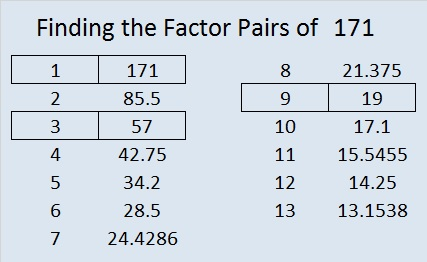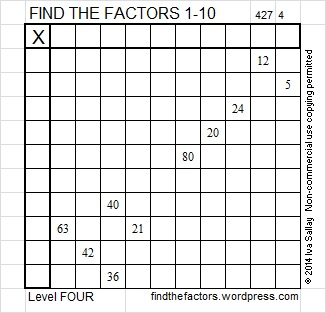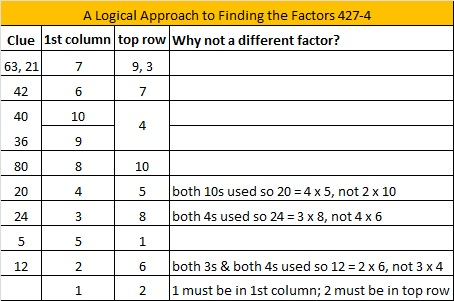# 171 and Level 4

 171 is a composite number. Factor pairs: 171 = 1 x 171, 3 x 57, or 9 x 19 Factors of 171: 1, 3, 9, 19, 57, 171 Prime factorization: 170 = 3 x 3 x 19, which can be written 170 = (3^2) x 19171 = 1 + 2 + 3 + 4 + 5 + 6 + 7 + 8 + 9 + 10 + 11 + 12 + 13 + 14 + 15 + 16 + 17 + 18 and is therefore a triangular number.

The fact that 171 is a triangular number is easy to recognize because 170 = 9 x 19 = (18/2) x 19. Any integer resulting from n(n + 1)/2 is a triangular number.Excel file of puzzles and previous week’s factor solutions: 10 Factors 2014-07-07This site uses Akismet to reduce spam. Learn how your comment data is processed.